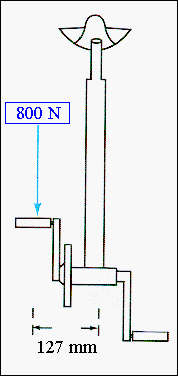Materials and Structure In addition to the compressive loading due to the weight of the rider, the seat tube experiences a moment due to the force applied to the pedals that is not along the center line of the seat tube and so is out of the plane of the frame. The diagram illustrates this force offset. Assuming that an 80 kg rider is standing on one pedal, the force is about 800 N and the offset from the tube center line is 127 mm to give a bending moment, M, acting on the tube of : M = (800 x 0.127) N.m = 101.6 N.m ~ 102 N.m.  For the circular cross-section tube, the stress caused by this moment will be a maximum in the material a distance D/2 from the centerline and will have a value: smax = MD/2I where I is the second moment of area of the tube. I = (p/64){D4 - d4}, where D and d are the outer and inner diameter of the tube respectively. For the head tube under consideration:                I = 1.1 x 10-8 m4, and this gives a maximum stress of 131 MPa. This is considerably larger than the stress due to the static loading (and very close to the yield stress of 180 MPa) and is determining in material selection and dimensions for this tube.From: McMahon and Graham: "The Bicycle and the Walkman," Merion (1992)